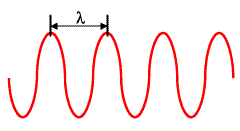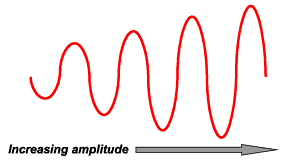Subscribe to our newsletter to receive the latest news and events from TWI:

# Properties of a sound wave relevant to ultrasonic testing

The main properties of a sound beam are:

• Wavelength
• Period
• Amplitude
• Frequency
• Velocity

Wavelength is defined as: the horizontal distance between any two successive equivalent points on the wave, and is usually represented by the symbol λ.Period is defined as: the time required for one complete cycle of the wave to pass by a point. One period is therefore the time it takes for a wave to travel a distance of one wavelength.

Amplitude is: the height of a wave and thus when there is a loud sound, the wave is high and the amplitude is large.A decibel is the unit that measures the intensity of sound and the softest sound that a human can hear is the zero point. When the sound is twice as loud, the decibel level goes up by six. Humans speak normally at 60 decibels.

### Frequency

Every cycle of sound has one condensation, a region of increased pressure, and one rarefaction, a region where air pressure is slightly less than normal. The frequency of a sound wave is measured in hertz. Hertz (Hz) indicate the number of cycles per second that pass a given location. If a speaker's diaphragm is vibrating back and forth at a frequency of 900 Hz, then 900 condensations are generated every second, each followed by a rarefaction, forming a sound wave whose frequency is 900 Hz.

How the brain interprets the frequency of an emitted sound is referred to as the pitch. The faster the vibrations the emitted sound makes (or the higher the frequency), the higher the pitch. Therefore, when the frequency is low, the sound is lower.